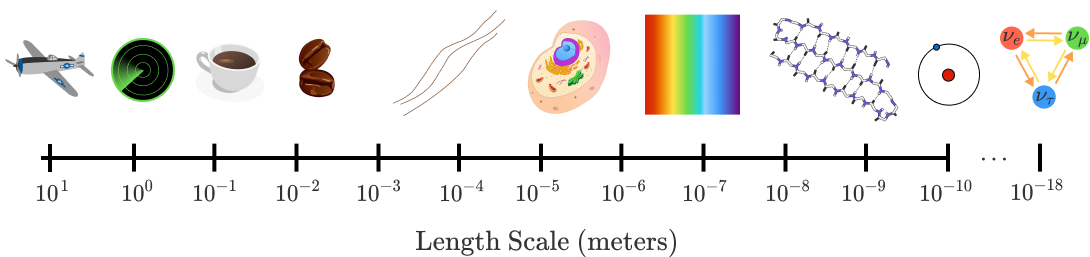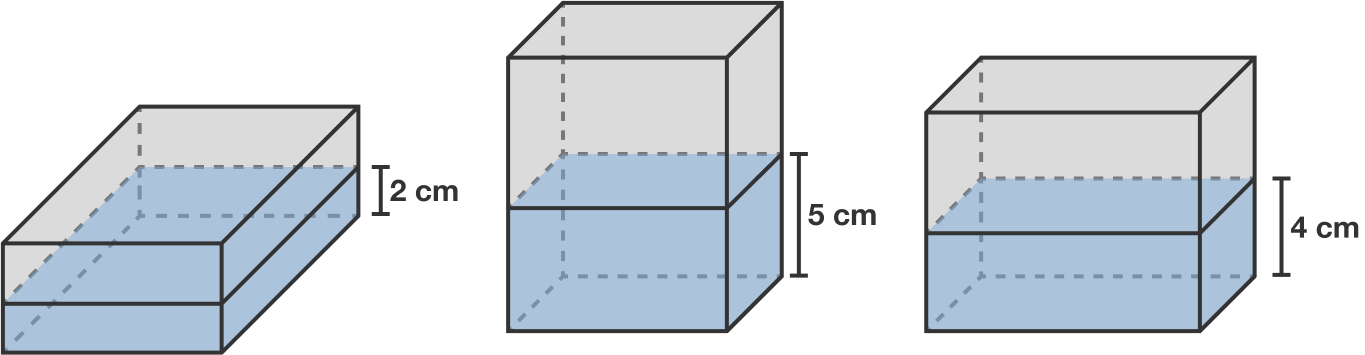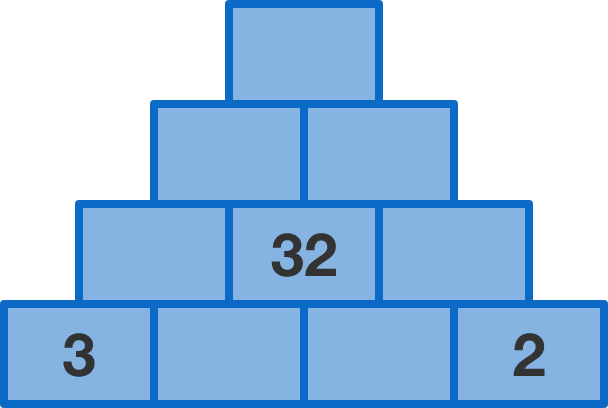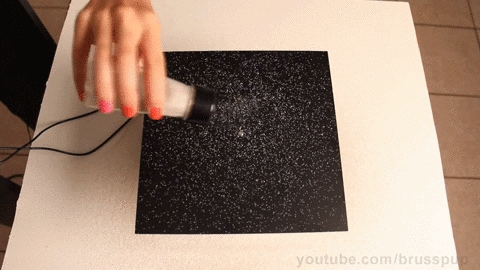# Problems of the Week

Contribute a problem

# 2018-06-11 BasicA rod rotates clockwise about its end 200 times per minute. This rod is kept in a dark room, and is flashed with bursts of light 201 times per minute.

How does the rod appear to move?

Note: The rate of the rod's rotation and the rate of the light flash are both constant.

The wavelength of radio waves is about $\SI{1}{\meter},$ while the wavelength of visible light is about $\SI{500}{\nano \meter}.$ As the wavelength of the waves gets smaller, their energy gets larger.When we look into a microscope, our eyes are detecting light waves scattered from the object we're looking at, just as a radar tower detects radio waves scattered from planes. But you can't see individual atoms with a microscope. This is because, as a rule, a wave won't scatter off an object much smaller than its wavelength. It would be like trying to track a speck of dust in the sky with a radar station.

Particle physicists use scattering to study subatomic particles a million times smaller than atoms.

What can we conclude about these scattering experiments?

A cuboid water tank is partially filled with $\SI{160}{\centi\meter}^3$ of water. Depending on which face it rests on, the height of water in the tank is $\SI{2}{\centi\meter}, \SI{5}{\centi\meter},$ or $\SI{4}{\centi\meter}.$

What is the volume of this tank $\big($in $\si{\centi\meter\cubed}\big)?$

Note: The diagram is not drawn to scale.The number on each brick is the sum of the numbers on the two bricks below it.

What number is on the top brick?

Powder is sprinkled on a plate. When the plate is vibrated at a certain frequency, the powder moves into a pattern.

What is happening where the powder gathers?Clip excerpted from the YouTube channel brusspup

×

Problem Loading...

Note Loading...

Set Loading...